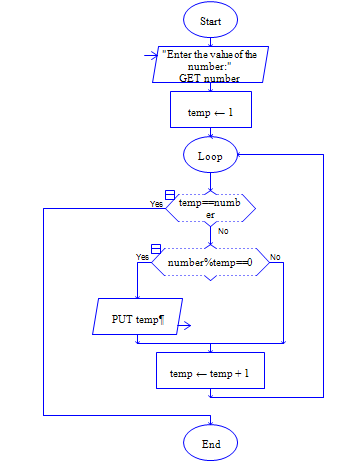# Program to print the Factors of a Number N

Sample Input 1 :

12

Sample Output 1:

1 2 3 4 6 12

#### Flow Chart Design#### Program or Solution

``` #include<stdio.h> int main() { int number, i; printf("Enter a Number: "); scanf("%d",&number); printf("Factors of %d: ", number); for(i=1; i <= number/2; ++i) { if (number%i == 0) { printf("%d ",i); } } printf("%d",number); return 0; } ```

#### Program Explanation

refer video tutorial